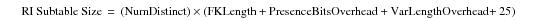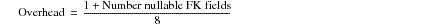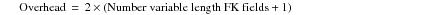# 15.00 - Reference Index Sizing Equation - Teradata Database

prodname
vrm_release
15.00
category
User Guide
featnum
B035-1094-015K

### Reference Index Sizing EquationIf fallback is defined for the child table in the relationship, then double the calculated result.

The following parameter definitions are used with this equation.

 Parameter Definition Row Count * 4 A count of the number of foreign key row references is stored in a reference index subtable. Each foreign key row count is 4 bytes long. FKLength The length of a fixed length foreign key value in bytes. Use one of these parameters depending on the reference index in question. If the FK column value is fixed, then use the length of the value. If the FK column value is variable, then use the average length of the variable length Foreign Key values. NumDistinct The value is an estimate of the number of distinct foreign key subtable values. Exclude null foreign keys from this estimate. Presence Bits Overhead Use the following equation to calculate this parameter:If there are no presence bits, then the value for this parameter is 0. VarLength Overhead Use the following equation to calculate this parameter:If there are no variable length foreign keys, then the value for this parameter is 0. RI Block Overhead Sum of the following factors. Row length RI row rowID Spare byte Presence octets Offsets Valid flag Foreign key count Reference array       = 25 bytes